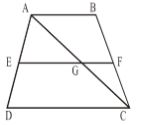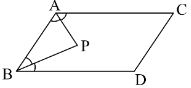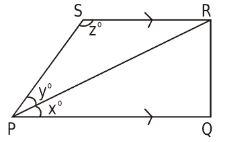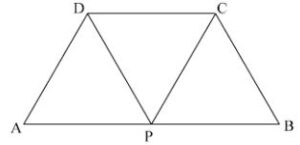Question: The diagonals do not necessarily bisect the interior angles at the vertices in a
(a) rectangle
(b) square
(c) rhombus
(d) all of these

Question: In figure, ABCD is a trapezium in which side AB is parallel to side DC and E is the midpoint of side AD. If F is a point on side BC such that the segment EF is parallel to side DC, then the value of EF is equal to(a) 1/4(AB + DC)
(b) 1/2(AB + DC)
(c) 1/3(AB + DC)
(d) 2/3(AB + DC)

Related: Time and work problems

Question: Three angles of a quadrilateral are 54º, 80º and 116º. Find the measure of the fourth angle.
(a) 70o
(b) 90o
(c) 110o
(d) 120o

Question: The sum of angles of a quadrilateral is _____ right angles.
(a) four
(b) two
(c) one
(d) none of these

Question: In the adjoining figure, AP and BP are angle bisectors of A and B which meet at P of a parallelogram ABCD. Then 2APB =(a) A + B
(b) A + C
(c) B + D
(d) C + D

Question: Is it possible to have a regular polygon with a measure of each exterior angle as 22º.
(a) Yes
(b) No

Related: Analogy quiz

Question: The no. of pairs of opposite angles of a quadrilateral is _____.
(a) 3
(b) 2
(c) 1
(d) 4

Question: How many sides have a regular polygon, each angle of which is of measure 108º?
(a) 6 sides
(b) 3 sides
(c) 4 sides
(d) 5 sides

Question: In the figure, ABCD is a trapezium in which AB = 7 cm, AD = BC = 5 cm, DC = x cm, and the distance between AB and DC is 4 cm. Find the value of x.(a) 12 cm
(b) 15 cm
(c) 13 cm
(d) none of these

Question: The diagonals do not necessarily intersect at right angles in a
(a) parallelogram
(b) rectangle
(c) rhombus
(d) kite

Related: Permutation questions

Question: What is the maximum exterior angle possible for a regular polygon?
(a) 120o
(b) 90o
(c) 130o
(d) 85o

Question: Determine the number of sides of a polygon whose exterior and interior angles are in the ratio 1 : 5.
(a) 12
(b) 13
(c) 14
(d) 15

Question: In the given figure,  If x = ⅗ y and y = 5/7 z then the values of x, y and z are(a) 60°,84°,36°
(b) 36°,84°,60°
(c) 36°,60°,84°
(d) 84°,60°,36°

Question: All kites are rhombuses. True or False?
(a) True
(b) False

Related: Probability questions

Question: Three angles of a quadrilateral are 30º, 150º and 100º. Find the fourth angle.
(a) 90o
(b) 70o
(c) 30o
(d) 80o

Question: The sides of a pentagon are produced in order and the exterior angles so obtained are of measure x°, (2x°), (3x + 10)°, (4x + 5)° and (5x°) respectively. Find the value of x
(a) 22
(b) 19
(c) 23
(d) 25

Question: In the adjoining figure, if ABCD is a square, the value of x is(a) 45°
(b) 60°
(c) 70°
(d) 30°

Question: Two adjacent angles of a parallelogram are equal. Find the measure of each angle of the parallelogram.
(a) 90o
(b) 80o
(c) 60o
(d) 70o

Related: Quant. aptitude examples

Question: A quadrilateral has all four angles of the same measure. What is the measure of each?
(a) 60°
(b) 70°
(c) 80°
(d) 90°

Question: Find the number of sides of a regular polygon, when each of its angles has a measure of 150°?
(a) 9
(b) 10
(c) 11
(d) 12

Question: In the adjoining figure, ABCD is a parallelogram. If AB = 2AD and P is the mid-point of AB, then CPD is equal to(a) 60°
(b) 90°
(c) 45°
(d) 120°

Question: If each interior angle of a regular polygon is 150º, how many sides does this polygon have?
(a) 12
(b) 9
(c) 10
(d) 11

Question: Find the length of the diagonal of a rectangle whose sides are 5 cm and 12 cm.
(a) 12 cm
(b) 13 cm
(c) 14 cm
(d) 15 cm

Question: Two angles of a quadrilateral are of measure 80º each. The other two angles are also equal. What is the measure of either of these two angles?
(a) 70°
(b) 80°
(c) 90°
(d) 100°

Question: The number of degrees at each exterior angle of a regular pentagon is
(a) 82º
(b) 60º
(c) 72º
(d) none of these

Question: RENT is a rectangle. Its diagonals meet at O. Find x, if OR = 3x + 5 and OT = 2x + 7.
(a) 1
(b) 2
(c) 8
(d) 5

Question: What is the measure of each angle of a regular hexagon?
(a) 125o
(b) 120o
(c) 110o
(d) 90o

Question: If the diagonals of a quadrilateral are perpendicular to each other, it is always a square. True or False?
(a) True
(b) False

Question: The interior angle of a regular polygon is 156º. The number of sides of the polygon is
(a) 15
(b) 12
(c) 18
(d) none of these

Question: Identity of all quadrilaterals that have four right angles
(a) Square and rectangle
(b) Square and rhombus

Question: The ratio between the exterior angle and the interior angle of a regular polygon is 2 : 7. Find the number of sides in the polygon.
(a) 8 sides
(b) 9 sides
(c) 10 sides
(d) 11 sides

Related: number series questions

Question: The two diagonals are not necessarily equal in a
(a) rectangle
(b) square
(c) rhombus
(d) isosceles trapezium

Question: How many sides does a regular polygon have if the measure of an exterior angle is 36°.
(a) 7
(b) 8
(c) 9
(d) 10